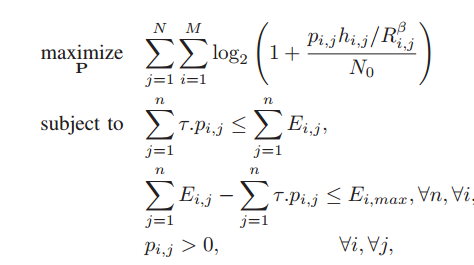# SINR maximization. NewbeeThis is maximization of SNR problem. how to write CVX code for this? Will nested For loop be used for double summation?
I am new to cvx. kindly help.

1 Like

You can use nested for loop in order to do multiple summation. However, by using elemenrtwiise ( `.*` and `./` ) calculations, you can instead use `sum(sum(log(matrix)))`. and avoid for loops. Declare `P` as a matrix variable with appropriate dimensions in order to do this.

``````% Input data
M = 2;
tow=2;
N = 10; %T=10 sec , tow=2sec so N=5
No=10^-15;
R = 50;
E=rand(M,N)*1000;
h=rand(M,N)*(-100);
Eimax= 37296;
x=0;
y=0;

for j=1:N
for i=1:M
cvx_begin
variable p
maximize sum(sum(log(1+(p(i,j)*h(i,j)/R)/No))/log(2))
subject to
x=x+sum(tow*p(:,j));
y=y+sum(rand(1,1)*1000);      % E = rand(1,1)*1000 // random addition of solar energy
x <= y;
y-x<=Eimax;
p(i,j)>0;
cvx_end
end
end
``````

I wrote this code.and this gives the following error.
x = evalin( ‘caller’, sprintf( '%s ', varargin{:} ) );
and it also gives error in the objective function. the cvx portion gives an optimal value and states that its inaccurate.

Kindly help with this.

1 Like

By having the for loops outside cvx_begin … cvx_end, you are solving `N*M` different optimization problems. You should not do You should declare
`variable p(M,N)`
and use `.*` so that log( …) winds up being an M by N matrix expression, to which you can apply sum(sum(…)) . You may need to brush up on the MATLAB basics.
Maybe something such as
`maximize(sum(sum(log(1+(p.*h/R)/No)/log(2)))`

Change `p(i,j)>0` to `p >= 0`

The scaling of your problem (input data) might not be too wonderful.

Your `x` and `y` constraints need to be fixed - I’ll let you figure that out. There may be other things wrong with or not good with your program, but this should get you started.

Thankyou I’ll make the changes

Can we exchange some questions about SINR?

@morgana If you have a specific question about implementing a convex optimization problem pertaining to SINR in CVX, then go ahead and ask it. For general discussion about SINR, please ask it somewhere other than this forum.